Lesson 19. LED BAR + MIC. LightMusic

• The purpose of this lesson

Hi! Today we will learn how to light multi-color led panels (only in the fire M5), relying on the components of the frequency of the audio signal received from the built-in (only in M5 fire) microphone.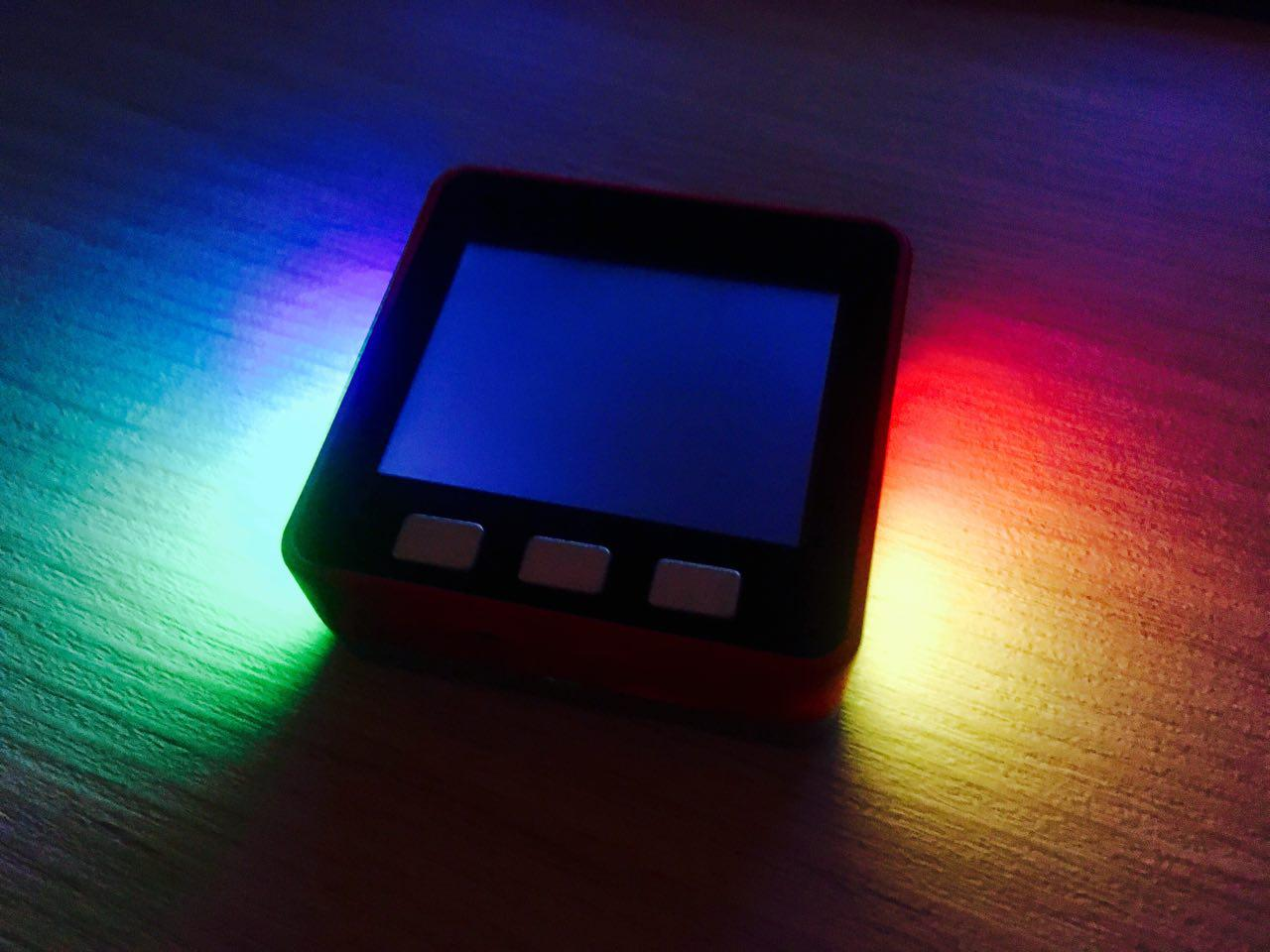Figure 1

This lesson will teach you how to connect and use third-party libraries to work with fast Fourier transform (FFT) and LEDs SK6818.

Short help

Color music is an art form based on a person's ability to associate sound sensations with light perceptions; this phenomenon in neurology is called synesthesia. Light music as an art is a derivative of music and is an integral part of it. Its purpose is to reveal the essence of music through visual perception. The main purpose of light music as an art is to study the ability of a person to experience the sensations imposed by light images when accompanied by music.

Music lovers have long noticed that musical instruments sound much louder and clearer in a well-lit room than in a darkened one. Therefore, when performing serious music, the light in the hall is usually not extinguished.

For the first time the connection between hearing and vision was very convincingly shown by Russian physicist and Russian physiologist academician P. P. Lazarev.

Details on Wiki: https://ru.wikipedia.org/wiki/Светомузыка

List of components for the lesson

• PC/MAC;
• M5STACK FIRE;
• USB-C cable from standard set.

Let's start!

Step 1. The installation for the library with Led work bar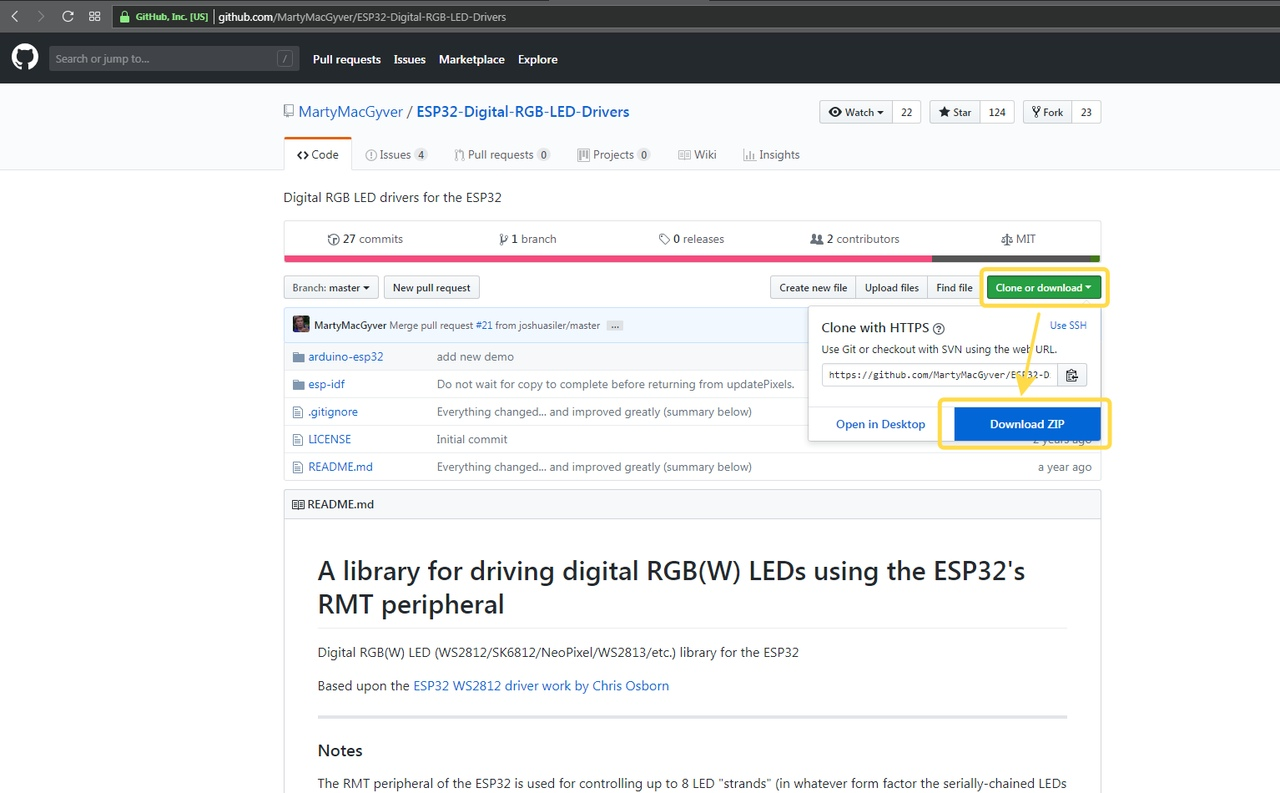Figure 2

Next, extract the archive to a new folder sketch and delete the file demo1.ino (Fig. 3).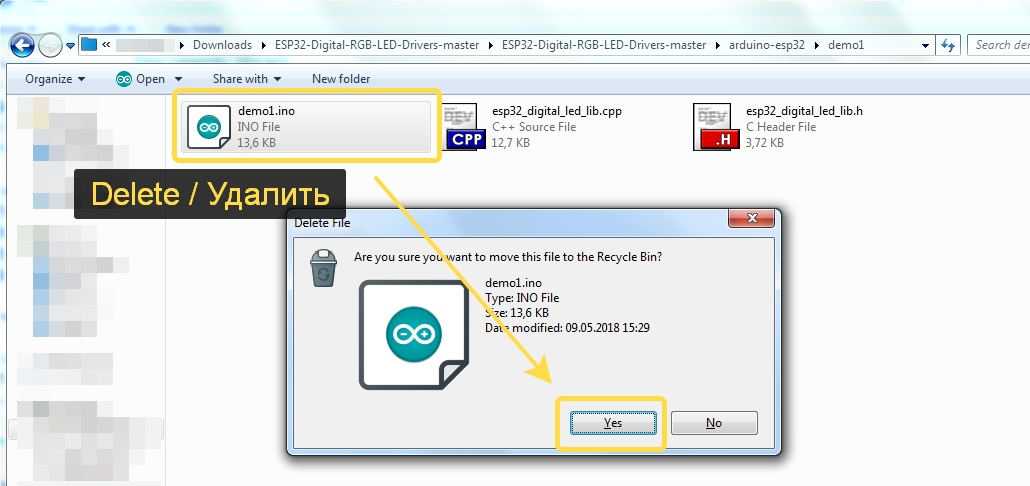Figure 3

Step 2. Installing the FFT library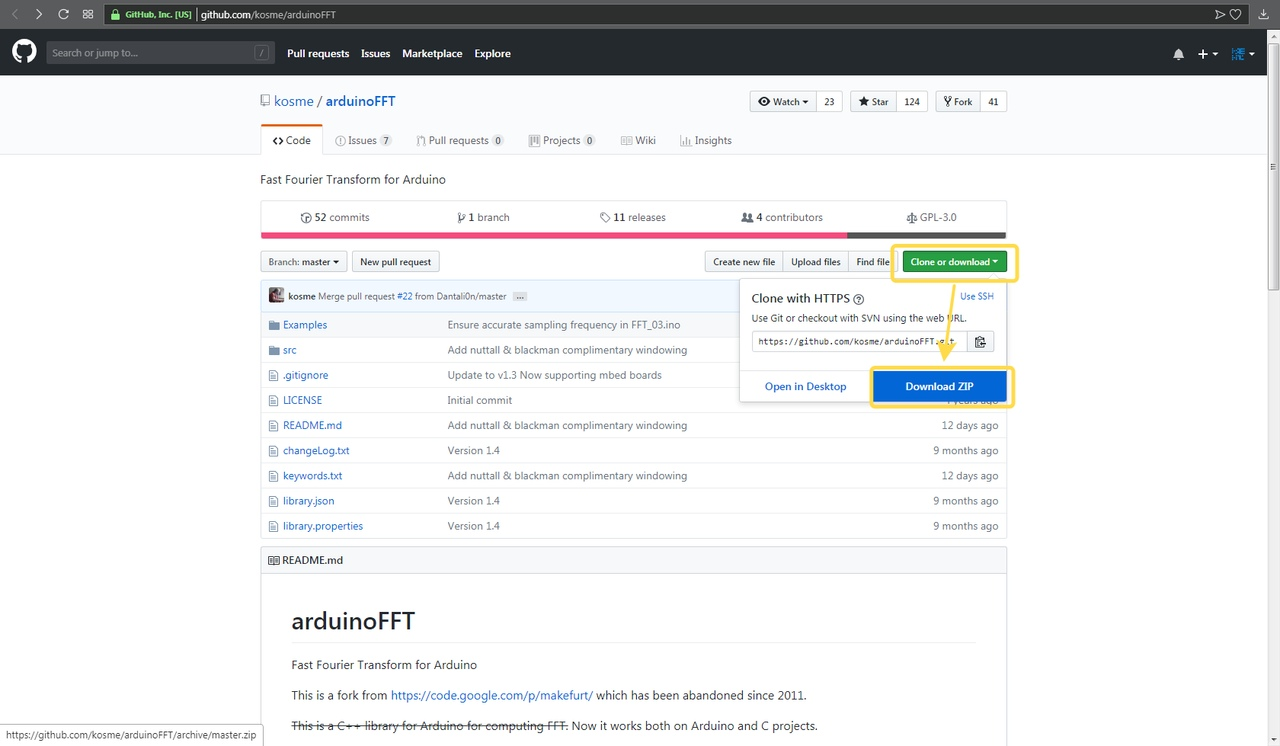Figure 4

In the extracted contents folder and Then copied C:\Users\USER_NAME\Documents\Arduino\libraries new name arduinoFFT is arduinoFFT-master (Fig. 5).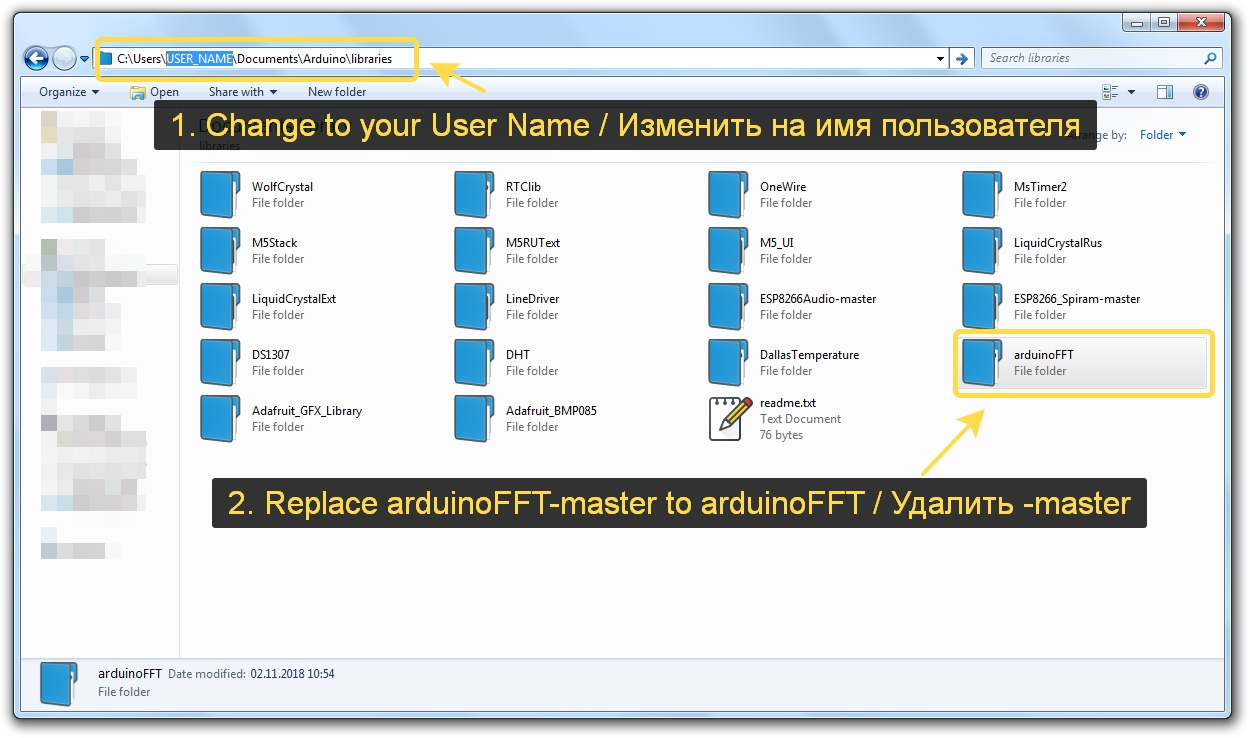Figure 5

Great! With libraries all :)

Step 3. Writing a sketch

Create a new sketch in the Arduino development environment and save it in the folder where the library files from step 1 are located (Fig. 6).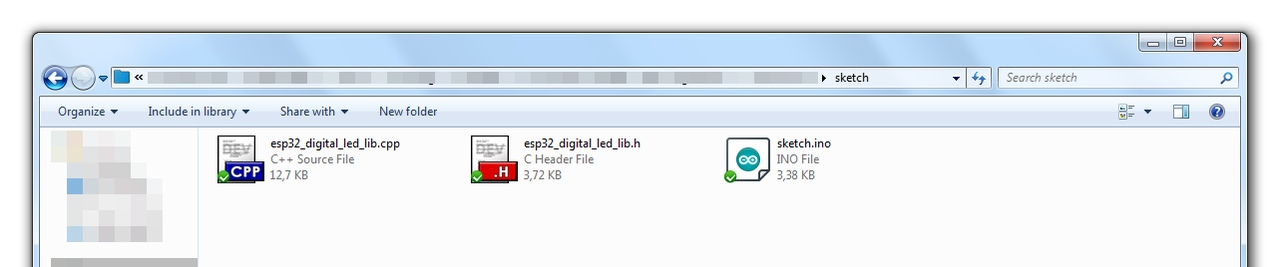Figure 6

Immediately connect the necessary libraries and create the necessary variables:

#include <M5Stack.h>
#include "arduinoFFT.h"
#include "esp32_digital_led_lib.h"
arduinoFFT FFT = arduinoFFT();

#define SAMPLES 256 //Must be a power of 2
#define SAMPLING_FREQUENCY 10000 //Hz, must be 10000 or less due to ADC conversion time. Determines maximum frequency that can be analysed by the FFT.
#define amplitude 50
unsigned int sampling_period_us;
unsigned long microseconds;
byte peak[] = {0,0,0,0,0,0,0};
double vReal[SAMPLES];
double vImag[SAMPLES];
unsigned long newTime, oldTime;
strand_t m_sLeds = {.rmtChannel = 0, .gpioNum = 15, .ledType = LED_WS2812B_V3, .brightLimit = 32, .numPixels = 10, .pixels = nullptr, ._stateVars = nullptr};

For simplified access to the LED BAR, I suggest using the following function void ledBar (int R, int G, int B, int M). Where int R, int G, int B - brightness of RED, GREEN and BLUE - respectively (from 0 to 255); int M - mode: from 0 to 9 - led number, 10 - all LEDs from the left panel, 11 - all LEDs from the right panel, 12 - all LEDs.

The LEDs are arranged clockwise (Fig. 7).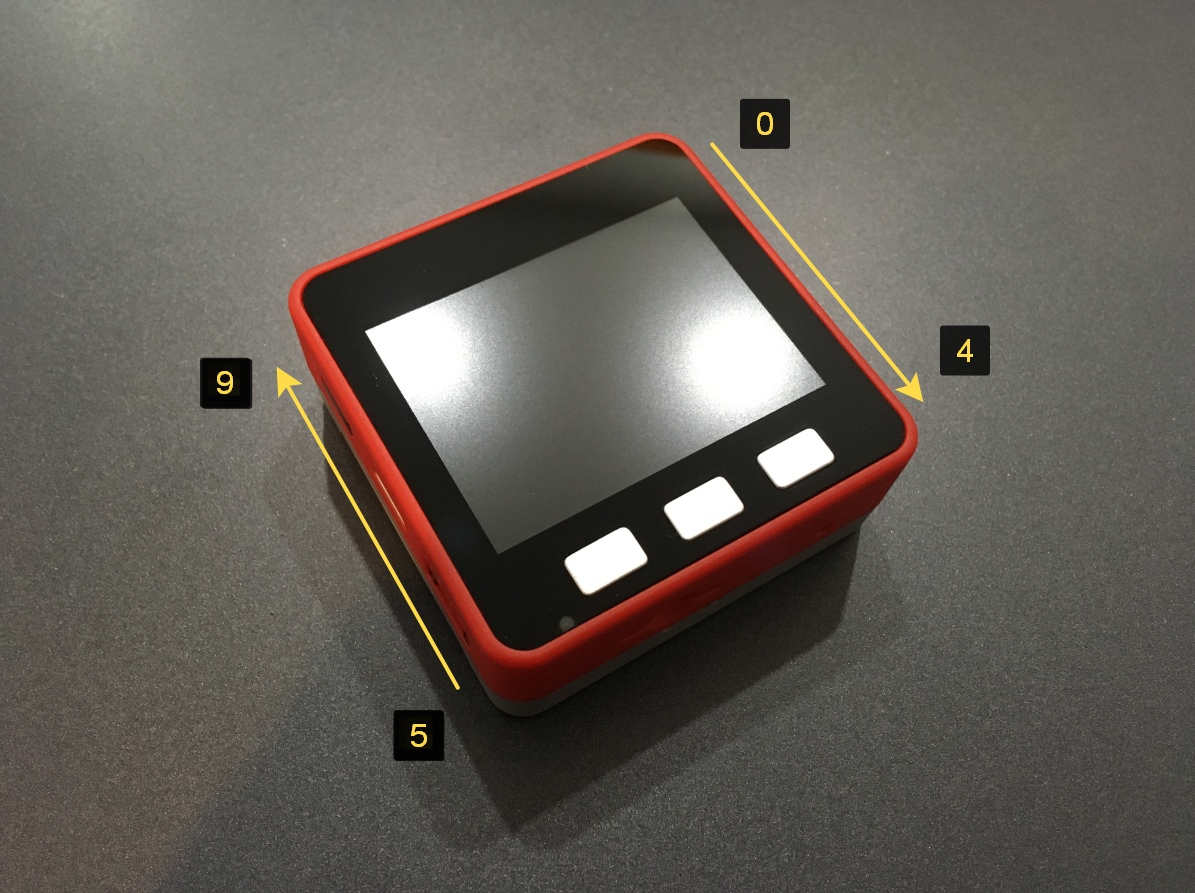Figure 7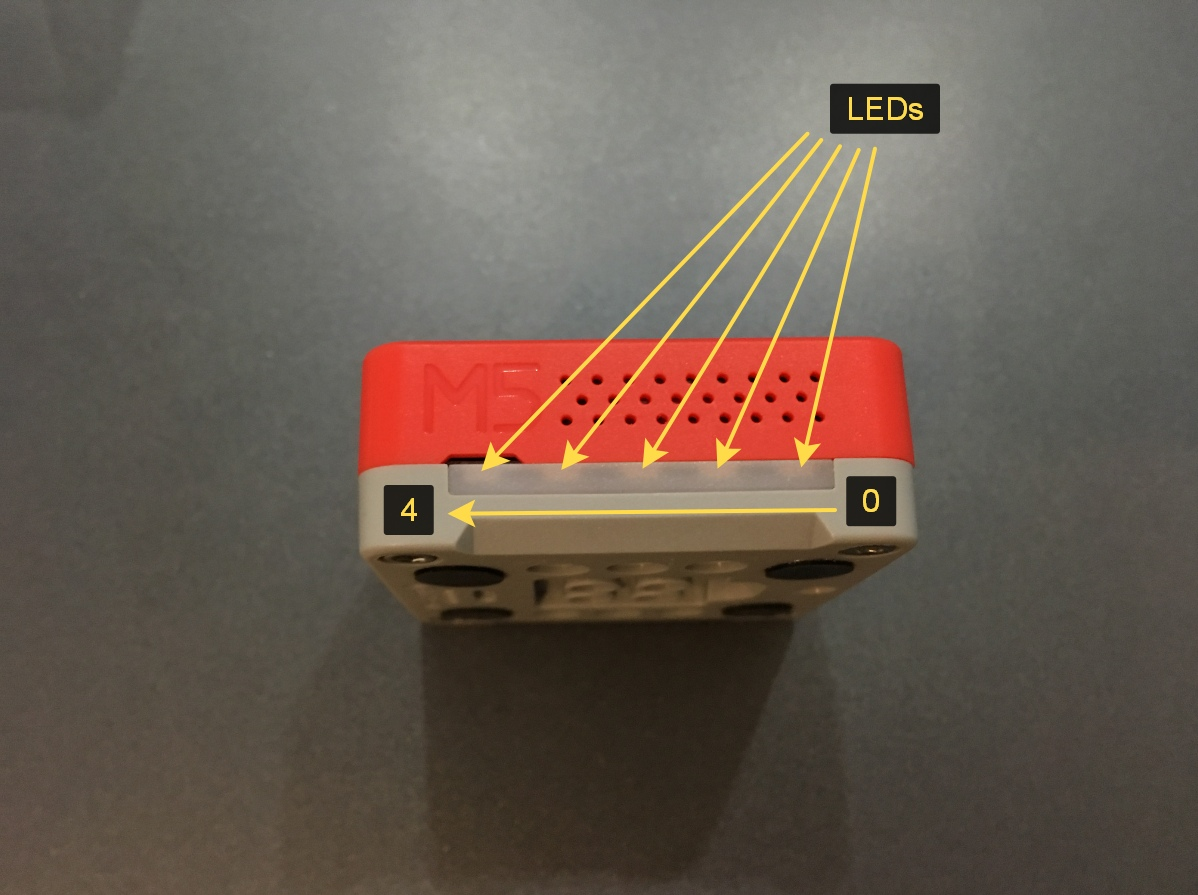Figure 7.1

void ledBar(int R, int G, int B, int M) {
if ((M < 0) || (M > 13)) return;
if (M == 11) // right
{
for (int i = 0; i < 5; i++)
{
m_sLeds.pixels[i] = pixelFromRGBW(R, G, B, 0);
}
}
else if (M == 10) // left
{
for (int i = 5; i < 10; i++)
{
m_sLeds.pixels[i] = pixelFromRGBW(R, G, B, 0);
}
}
else if (M == 12) // all
{
for (int i = 0; i < 10; i++)
{
m_sLeds.pixels[i] = pixelFromRGBW(R, G, B, 0);
}
}
else
{
m_sLeds.pixels[M] = pixelFromRGBW(R, G, B, 0);
}
digitalLeds_updatePixels(&m_sLeds);
}

Main part. Do not forget about void dacWrite(25, 0); that the speaker does not make strange sounds and cod.

void setup(){
M5.begin();
pinMode(25, OUTPUT);
pinMode(34, INPUT);
sampling_period_us = round(1000000 * (1.0 / SAMPLING_FREQUENCY));
pinMode(15, OUTPUT);
digitalWrite (15, LOW);
if (digitalLeds_initStrands(&m_sLeds, 1)) {
Serial.println("Can't init LED driver().");
}
digitalLeds_resetPixels(&m_sLeds);
}

Note that pinMode (34, INPUT) is the analog input to which the built-in microphone is connected (Fig. 8) through the amplifier, so set it to INPUT.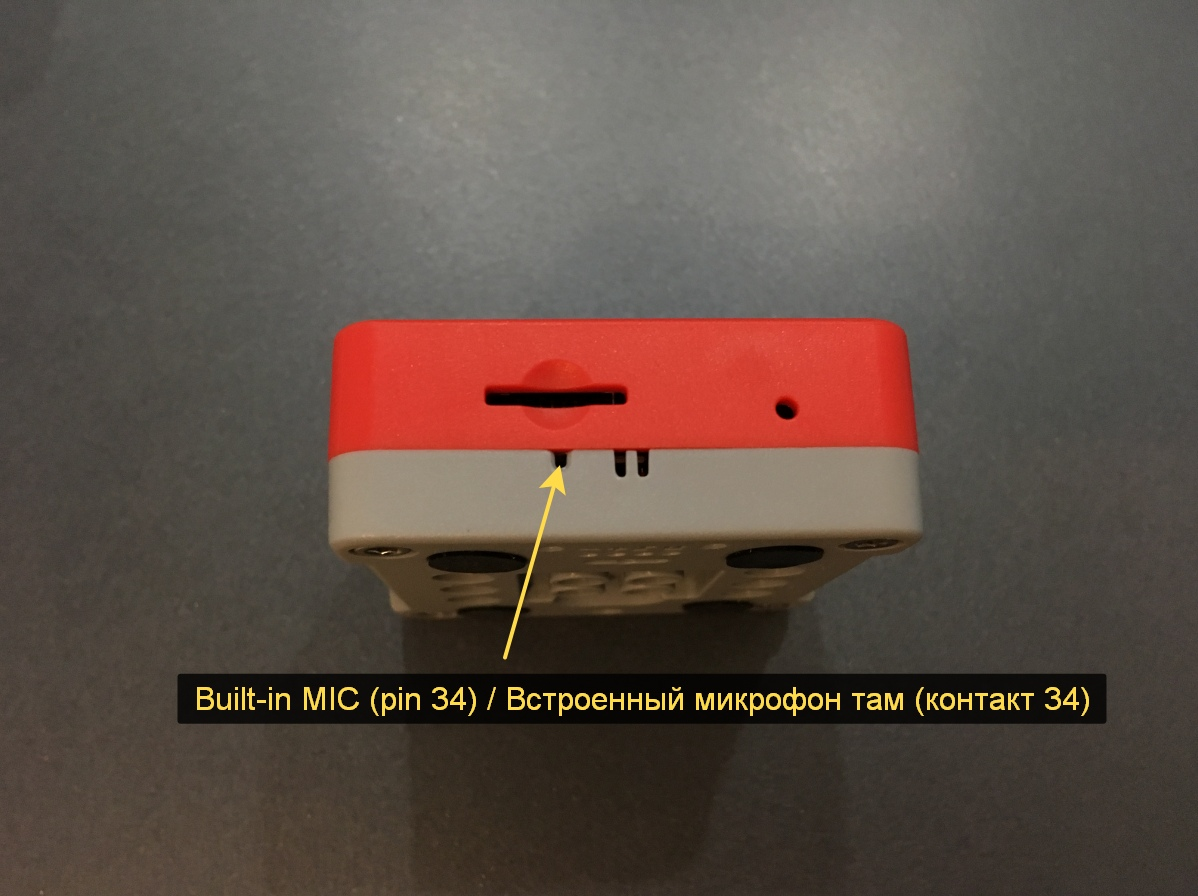Figure 8

void loop() {
for (int i = 0; i < SAMPLES; i++) {
newTime = micros() - oldTime;
oldTime = newTime;
vReal[i] = analogRead(34); // A conversion takes about 1mS on an ESP8266
vImag[i] = 0;
while (micros() < (newTime + sampling_period_us));  // do nothing to wait
}

FFT.Windowing(vReal, SAMPLES, FFT_WIN_TYP_HAMMING, FFT_FORWARD);
FFT.Compute(vReal, vImag, SAMPLES, FFT_FORWARD);
FFT.ComplexToMagnitude(vReal, vImag, SAMPLES);
dacWrite(25, 0);

for (int i = 2; i < (SAMPLES/2); i++){ // Don't use sample 0 and only first SAMPLES/2 are usable. Each array eleement represents a frequency and its value the amplitude.
if (vReal[i] > 200) { // Add a crude noise filter, 4 x amplitude or more
if (i<=5 )             displayBand(0,(int)vReal[i]/amplitude); // 125Hz
if (i >5   && i<=12 )  displayBand(1,(int)vReal[i]/amplitude); // 250Hz
if (i >12  && i<=32 )  displayBand(2,(int)vReal[i]/amplitude); // 500Hz
if (i >32  && i<=62 )  displayBand(3,(int)vReal[i]/amplitude); // 1000Hz
if (i >62  && i<=105 ) displayBand(4,(int)vReal[i]/amplitude); // 2000Hz
if (i >105 && i<=120 ) displayBand(5,(int)vReal[i]/amplitude); // 4000Hz
if (i >120 && i<=146 ) displayBand(6,(int)vReal[i]/amplitude); // 8000Hz
}
}
}

Light the LEDs will be using the function void displayBand (int band, int dsize). Where band - frequency in the signal; dsize - number of frequency in the signal. You can safely experiment here and achieve the best flashes.

void displayBand(int band, int dsize){
if (band > 1) dsize += 100;
if (dsize >= 150)
{
ledBar(0, 0, 0, 12);
if (band == 0)
{
if (dsize >= 300)
{
ledBar(255, 0, 0, 0);
ledBar(255, 0, 0, 1);
}
}
else if (band == 1)
{
if (dsize >= 180)
{
ledBar(255, 255, 0, 3);
ledBar(255, 255, 0, 4);
}
}
else if (band == 2)
{
if (dsize >= 170)
{
ledBar(0, 255, 0, 5);
ledBar(0, 255, 0, 6);
}
}
else
{
ledBar(0, 0, 255, 8);
ledBar(0, 0, 255, 9);
}
}
}

Final step

That's all :)